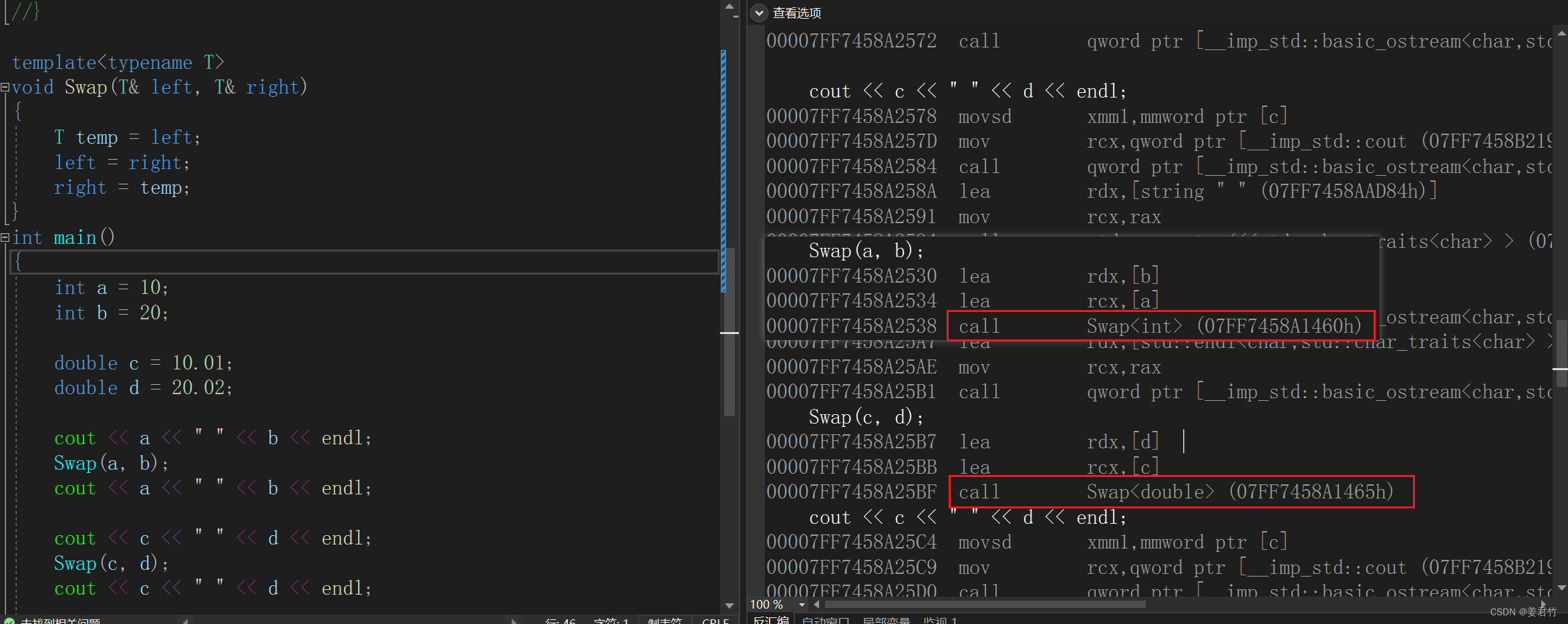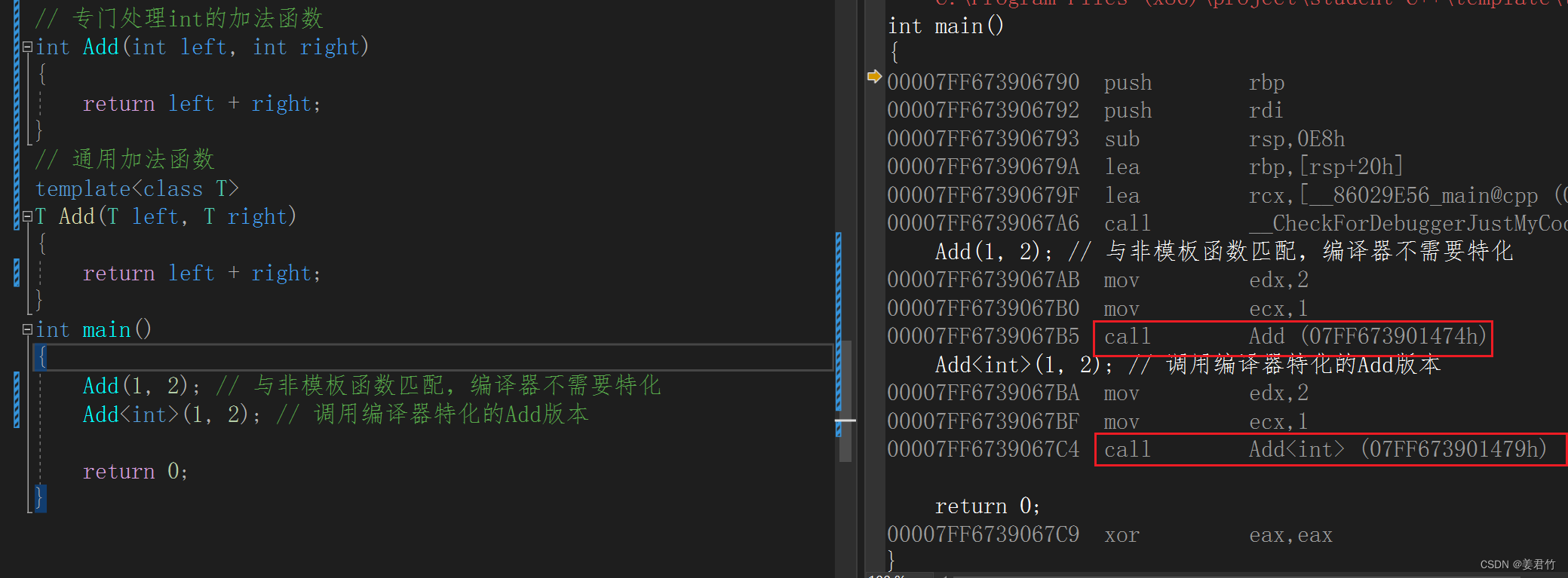## 【C++】模板初阶

article2023/12/11 13:06:16/

• 泛型编程
• 函数模板
• 概念
• 格式
• 实例化
• 匹配原则
• 类模板
• 定义格式
• 实例化

### 泛型编程

当我们的一个函数涉及到多个类型的处理时，我们就需要重载函数来实现，但是重载函数是存在一些局限性的。
重载函数仅仅是类型不同，代码的复用率较低，只要有新类型出现，就需要用户自己增加对应的函数。并且代码的可维护性低，一个出错可能会导致所有的重载均出现错误。

void Swap(int& left, int& right)
{
int temp = left;
left = right;
right = temp;
}
void Swap(double& left, double& right)
{
double temp = left;
left = right;
right = temp;
}
int main()
{
int a = 10;
int b = 20;

double c = 10.01;
double d = 20.02;

cout << a << " " << b << endl;
Swap(a, b);
cout << a << " " << b << endl;

cout << c << " " << d << endl;
Swap(c, d);
cout << c << " " << d << endl;

return 0;
}


所以我们需要进行泛型编程，泛型编程是指编写与类型无关的通用代码，这是代码复用的一种手段。而模板是泛型编程的基础。
模板就是告诉编译器一个模子，让编译器基于这个模板根据不同的类型生成代码。这样就提高了代码的复用率也提高了可维护性。模板分为函数模板和类模板。

### 函数模板

#### 概念

函数模板代表了一个函数家族，该函数模板与类型无关，在使用时被参数化，根据实参类型产生函数的特定类型版本。

#### 格式

template<typename T1, typename T2, ……, typename Tn> 函数。

template<typename T>
void Swap(T& left, T& right)
{
T temp = left;
left = right;
right = temp;
}
int main()
{
int a = 10;
int b = 20;

double c = 10.01;
double d = 20.02;

cout << a << " " << b << endl;
Swap(a, b);
cout << a << " " << b << endl;

cout << c << " " << d << endl;
Swap(c, d);
cout << c << " " << d << endl;

return 0;
}


函数模板是一个蓝图，它本身并不是函数，是编译器用使用方式产生特定具体类型函数的模具。所以模板就是将本来应该我们做的重复的事情交给了编译器。
在编译器编译阶段，对于模板函数的使用，编译器需要根据传入的实参类型来推演生成对应类型的函数以供调用。### 实例化

用不同类型的参数使用函数模板时，称为函数模板的实例化。模板参数实例化分为：隐式实例化和显示实例化。
隐式实例化是让编译器根据实参推演模板参数的实际类型。

template<typename T>
T Add(const T& num1, const T& num2)
{
T sum = num1 + num2;
return sum;
}
int main()
{
int a = 10;
int b = 20;

double c = 10.01;
double d = 20.02;
// 隐式实例化时，如果参数的类型不一样会报错
// 解决办法有两种，强转数据类型或者显示实例化
cout << Add(a, (int)c) << endl;

cout << Add((double)b, d) << endl;

return 0;
}


显示实例化是在函数名后面用<>指定模板参数的实际类型。

template<typename T>
T Add(const T& num1, const T& num2)
{
T sum = num1 + num2;
return sum;
}
int main()
{
int a = 10;
int b = 20;

double c = 10.01;
double d = 20.02;
// 显示实例化
cout << Add<int>(a, c) << endl;
cout << Add<double>(a, c) << endl;

return 0;
}


#### 匹配原则

一个非模板函数可以和一个同名的函数模板同时存在，而且该函数模板还可以被实例化为这个非模板函数。

// 专门处理int的加法函数
{
return left + right;
}
// 通用加法函数
template<class T>
{
return left + right;
}
int main()
{

return 0;
}对于非模板函数和同名函数模板，如果其他条件都相同，在调用时会优先调用非模板函数而不会从该模板生出一个实例。如果模板可以产生一个具有更好匹配的函数，那么将选择模板。

// 专门处理int的加法函数
{
return left + right;
}
// 通用加法函数
template<class T>
{
return left + right;
}
int main()
{

return 0;
}


模板函数不允许自动类型转换，但普通函数可以进行自动类型转换。

### 类模板

#### 定义格式

template<class T1, class T2, …, class Tn>
class 类模板名
{
类内成员定义
};

// 动态顺序表
// 注意：Vector不是具体的类，是编译器根据被实例化的类型生成具体类的模具
template<class T>
class Vector
{
public:
Vector(size_t capacity = 10)
: _pData(new T[capacity])
, _size(0)
, _capacity(capacity)
{}

// 使用析构函数演示：在类中声明，在类外定义。
~Vector();

void PushBack(const T& data)；
void PopBack()；
// ...

size_t Size() { return _size; }

T& operator[](size_t pos)
{
assert(pos < _size);
return _pData[pos];
}

private:
T* _pData;
size_t _size;
size_t _capacity;
};
// 注意：类模板中函数放在类外进行定义时，需要加模板参数列表
template <class T>
Vector<T>::~Vector()
{
if (_pData)
delete[] _pData;
_size = _capacity = 0;
}


#### 实例化

类模板实例化与函数模板实例化不同，类模板实例化需要在类模板名字后加上<数据类型>，类模板名字不是真正的类，实例化之后的结果才是真正的类。

// Vector类名，Vector<int>才是类型
Vector<int> s1;
Vector<double> s2;


### π-Day快乐：Python可视化π

π-Day快乐&#xff1a;Python可视化π 今天是3.14&#xff0c;正好是圆周率 π\piπ 的前3位&#xff0c;因此数学界将这一天定为π\bold{\pi}π day。 π\piπ 可能是最著名的无理数了&#xff0c;人类对 π\piπ 的研究从未停止。目前人类借助计算机已经计算到 π\piπ 小数…

### 量化(1):基础知识

1. Tops的含义 1TOPS代表处理器每秒可进行一万亿次（10^12）操作 2. 定点数 2.1 定点数的含义 大家都知道，数字既包括整数，又包括小数，如果想在计算机中，既能表示整数，也能表示小数，关键就在于这个小数点如何表示？ 计算机科学家们想出一种方法，即约定计算机中小数…

### 裸辞3个月，面试了25家公司，终于找到心仪的工作了

​上半年裁员&#xff0c;下半年裸辞&#xff0c;有不少人高呼裸辞后躺平真的好快乐&#xff01;但也有很多人&#xff0c;裸辞后的生活五味杂陈。 面试25次终于找到心仪工作 因为工作压力大、领导PUA等各种原因&#xff0c;今年2月下旬我从一家互联网小厂裸辞&#xff0c;没…